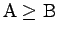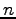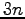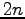Sequential Circuit Design

Tom Kelliher, CS 240

Apr. 9, 2010

Announcements

Collect assignment.

Assignment

New written assignment.

From Last Time

Sequential circuit analysis.

Outline

1. Sequential circuit design process.

2. Unused states.

3. Examples.

Coming Up

VHDL for sequential circuits.

Sequential Circuit Design Process

1. Obtain a state diagram. Assign binary numbers to the states (a non-trivial problem, actually).

2. Obtain a state table.

3. Derive flip-flop input equations from the next state entries and output equations. Simplify.

Unused States

Suppose your design has 6 states:

1. Two unused states.

2. What happens if the circuit enters one of these states?

Examples

1. Sequence recognizer for 010.

2. Serial comparator. Inputs: A, B, msb. A and B are received least significant bit first. Receipt of msb is co-incident with msb's of A and B and resets circuit to begin next comparison. Output 0 if, otherwise 1.

3. Serial comparator. Inputs: A, B, lsb. A and B are received most significant bit first. Receipt of lsb is co-incident with lsb's of A and B and resets circuit to begin next comparison. Output 0 if, otherwise 1.

4. Given an unsigned binary valueserially, starting from the lsb, compute. Hint: How would you compute?

Thomas P. Kelliher 2010-04-08
Tom Kelliher